### Mensuration - Worksheets

CBSE Worksheet 01

Ch-10 Mensuration
1. The side of a square is 12 m. Its perimeter is
1. 144 m
2. 48 m
3. 24 m
4. 36 m
2. The shape of your class room blackboard is
1. circle
2. equilateral triangle
3. square
4. rectangle
3. Which figure encloses more area: a square of side 2 cm ; a rectangle of side 3 cm and 2 cm ;An equilateral triangle of side 4 cm?
1. None of these
2. square
3. equilateral triangle
4. rectangle
4. The perimeter of the given figure is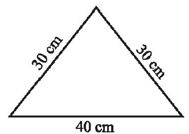1. 70 cm
2. None of these
3. 60 cm
4. 100 cm
5. The area of square is 100 $\text{c}{\text{m}}^{\text{2}}$. Its side is
1. 5 cm
2. 10 cm
3. 20 cm
4. 10.5 cm
6. Match the following:-
ShapesPerimeter
a.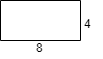i. 15
b.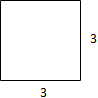ii. 8
c.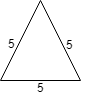iii. 24
d.iv. 12
7. Fill in the blanks:-
1. _____ is a rectangle whose all sides are equal.
2. The amount of surface enclosed by a figure is called its ____.
3. For fencing the plot, we need to calculate its _____.
4. _____ is the sum of all sides.
8. State True or False:-
1. For tiling a rectangular Plot, we must calculate its area.
2. Length and breadth of a Rectangle are 1.5m and 1m. Area is 1.5m2.
3. The Perimeter of a square is 4 times the length of the side.
4. To find the length of fencing the square playground we must find its Perimeter.
9. Find the area of the rectangle whose side are :3 cm and 4 cm
10. Find the area of the rectangle whose side are :12 m and 21 m
11. Find the perimeter of each of the following figures given below?
1.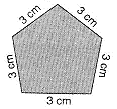2.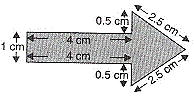3.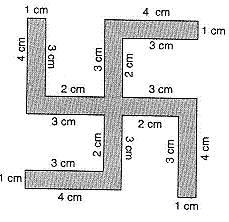12. Find the perimeter of the following figure?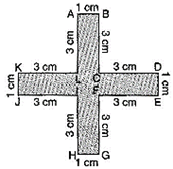13. Find the perimeter of the following figure given below.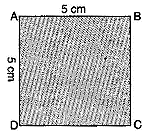14. Find the area of the following figure: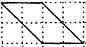15. The length of rectangle is thrice its breadth and its perimeter is 48cm. Find length and breadth of rectangle.

CBSE Worksheet 01
Ch-10 Mensuration

1. (b) 48 m, Explanation: Perimeter of square = $4l$
here length = 12 m
so, the perimeter = 4 * 12
= 48 m
1. (d) rectangle, Explanation: Shape of the blackboard in class room is rectangle as the length of the blackboard is more than the breadth it.
1. (c) equilateral triangle, Explanation: Area of square = $l×l$ , when l = 2 cm , Area = 4 cm2
Area of rectangle = $l×b$ , when l = 3 cm and b = 2 cm , Area =$3×2$ = 6 cm2
Area of an equilateral triangle =$\left(\sqrt{3}/4\right){a}^{2}$ , when a = 4 cm , Area = 6.93 cm2
so, the area of eqilateral traiangle is more
1. (d) 100 cm, Explanation: Perimeter means addition of all sides.
So Perimeter of given triangle = 30 + 30 + 40 = 100 cm
1. (b) 10 cm, Explanation: Area of square = $l\ast l$ here area = 100 $\text{c}{\text{m}}^{\text{2}}$ so the lenght is = $l=\sqrt{1}00$ length = 10 cm
1. $\to$ iii
2. $\to$ iv
3. $\to$ i
4. $\to$ ii
1. Square
2. area
3. Perimeter
4. Perimeter
1. True
2. True
3. True
4. True
1. Area of the rectangle = Length × Breadth = 3 × 4 cm = 12 sq cm
2. Area of the rectangle = Length × Breadth = 12 m × 21 m = 252 sq m
1. Perimeter = 3 cm + 3 cm + 3 cm + 3 cm + 3 cm
= 15 cm
2. Perimeter = 1 cm + 4 cm + 0.5 cm + 2.5 cm + 2.5 cm + 0.5 cm + 4 cm
= 15 cm
3. Perimeter = 1 cm + 3 cm + 2 cm + 3 cm + 4 cm + 1 cm + 3 cm + 2 cm + 3 cm + 4 cm + 1 cm + 3 cm + 2 cm + 3 cm + 4 cm + 1 cm + 3 cm + 2 cm + 3 cm + 4 cm
= 52 cm
3. Perimeter
= AB + BC + CD + DE + EF + FG + GH + HI + IJ + JK + KL + LA
= 1 cm + 3 cm + 3 cm + 1 cm + 3 cm + 3 cm + 1 cm + 3 cm + 3 cm + 1 cm + 3 cm + 3 cm
= 28 cm
4. Perimeter
= AB + BC + CD + DA
= 5 cm + 5 cm + 5 cm + 5 cm = 20 cm
5. Full-filled squares = 2
half-filled squares = 4
Area covered by full squares = 2 $×$ 1 sq unit = 2 sq units
Area covered by half squares = 4 $×$ $\frac{1}{2}$ sq unit = 2 sq units
$\therefore$ Total Area = 2 sq units + 2 sq units = 4 sq units
6. Let the breadth of rectangle = b
length of rectangle = 3b
Perimeter of rectangle $=2×\left(3b+b\right)$
$48=2×\left(3b+b\right)$
$\frac{48}{2}=4b$
24 = 4b
$\frac{24}{4}=b$
$⇒6=b$ breadth = 6cm
length = 3b